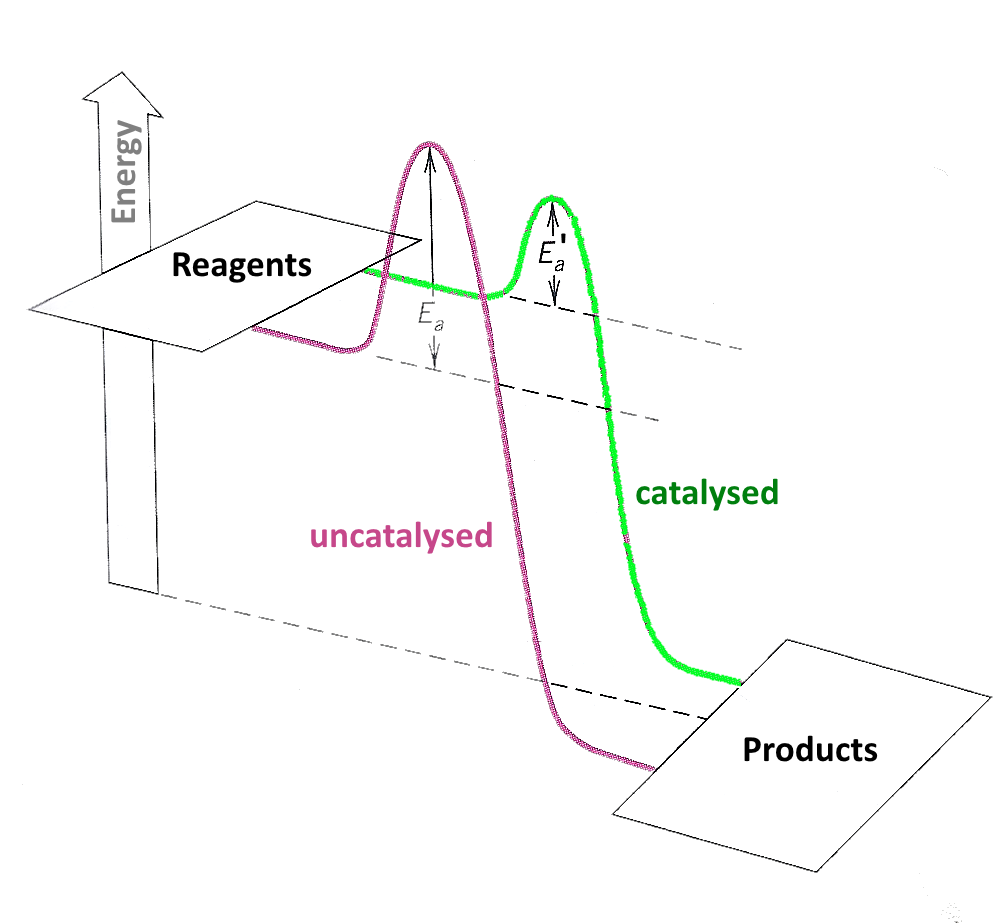Search:

# Activation energy and catalysis

## Reminder

The →       activation energy $E_a$ of a reaction determines its rate constant $k$: $k$ $=$ $Ae^{-\frac{E_a}{R\cdot T}}$

where - $T$ is the Kelvin temperature (expressed in $K$) - $E_a$ l'énergie d'activation (expressed in $J$ ) - $R$ is the ideal gas constant $(8,3 \frac{J}{mol\; K} )$ - $A$ $\approx$ a constant for a given reaction

## Action of a catalystA catalyst lowers the activation energy

If $E_a$ decreases, $k$ increases and thus the reaction rate:(1) shows the evolution of the molarity of an reagent $A$ without, (2) with a catalyst.